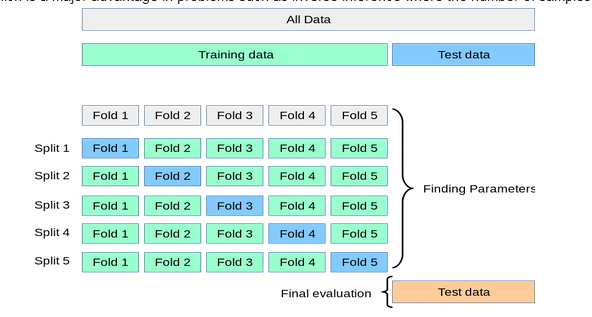# 关于python：如何使用交叉验证来检测过度拟合：差异阈值应为多少？

## How to detect overfitting with Cross Validation: What should be the difference threshold?

 1234567891011121314 Split Number  1 Accuracy= 0.9454545454545454 Precision= 0.94375 Recall= 1.0 Split Number  2 Accuracy= 0.9757575757575757 Precision= 0.9753086419753086 Recall= 1.0 Split Number  3 Accuracy= 0.9695121951219512 Precision= 0.9691358024691358 Recall= 1.0

 123 Accuracy= 0.9530201342281879 Precision= 0.952054794520548 Recall= 1.012345 from sklearn.model_selection import cross_validate from sklearn.metrics import recall_score scoring = ['precision_macro', 'recall_macro'] clf = svm.SVC(kernel='linear', C=1, random_state=0) scores = cross_validate(clf, iris.data, iris.target, scoring=scoring,cv=5)

return_train_score is set to False by default to save computation
time. To evaluate the scores on the training set as well you need to
be set to True